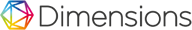### PERFORMANCE OF TRAPEZOIDAL METHOD AND GAUSS-LEGENDRE METHOD IN SETTLEMENT OF CERTAIN INTEGRAL ASSISTED MATLAB#### AbstractThis study aimed to compare the performance of a particular integral calculation using the Trapezoid and Gauss-Legendre method in five integral cases to determine the accuracy of the integral results value of both methods which are based on the relative error percentage. This study is a literature research which formulate mathematical problem that can be solved with the usual arithmetic operations or calculations which are addition, subtraction, multiplication and division to obtain numeral with the best accuracy. Trapezoidal method formula used is while the Gauss-Legendre formula used is ,
This study shows that the Gauss-Legendre method has better performance by giving the percentage of error which is relatively better than Trapezoid method used in the five cases.

Keywords: Numerical Methods, Trapezoid method, the method of Gauss-Legendre

#### Full Text:

PDF

DOI: https://doi.org/10.26486/mercumatika.v1i1.189

#### Article Metrics

Abstract view : 604 times
PDF - 1303 times

### Refbacks

• There are currently no refbacks.

## Jurnal Mercumatika : Jurnal Penelitian Matematika dan Pendidikan Matematika Indexed by

####